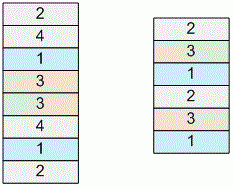Competitions

# Bitris

There is a set of 2 × n cubes. Each cube has an integer ranging from 1 to n assigned to it, labeling each of the cube's sides. Each number is written on exactly two cubes. Cubes are placed one on top of another, in a pile. If two cubes with the same number stand next to each other, they annihilate: both cubes disappear, and cubes standing above come down to fill the space.

Your task is to disassemble the pile – eliminate all cubes. You are allowed to swap any two neighboring cubes. A swap could be done only after all possible annihilations are done.For example, if n = 4 and cubes are standing as you see on the left then you need to make one swap. Cubes with label 3 annihilate immediately; you swap the fourth bottom cube (with label 1) and the fifth bottom cube (with label 4); afterwards, cubes with labels 4 annihilate, followed by cubes with labels 1 and labels 2. Other option is to swap third and fourth bottom cubes (in this case cubes with labels 1 and 4 annihilate at same time, followed by cubes with label 2), or second and third.

If n = 3, and cubes are standing like shown on the right, you need to perform 3 swaps. One way to solve is to swap fifth and sixth cubes, then fourth and fifth; cubes with labels 2 annihilate; then swap second and third; other cubes annihilate simultaneously.

The task is to find the minimal number of swaps, such that all cubes are eliminated.

#### Input

The first line contains the positive integer n (2n100000). The second line contains the labels of all cubes, bottom up, split by spaces.

#### Output

Print the minimal number of swaps necessary to destroy all cubes.

Time limit 1 second
Memory limit 128 MiB
Input example #1
4
2 1 4 3 3 1 4 2

Output example #1
1

Input example #2
3
1 3 2 1 3 2

Output example #2
3

Input example #3
3
1 3 2 2 3 1

Output example #3
0

Source 2012 Southeastern Europe Regional Contest, Bucharest, Vinnica, October 13, Problem B